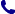• Kontakt
• Newsletter Zapisz się po informacje o nowościach i specjalnych ofertach. Administrator danych: ABE-IPS sp. z o.o.
Wybierz interesujące Cię kategorie
Wyrażam zgodę na przetwarzanie moich danych osobowych w celach marketingowych, tym samym akceptuję Regulamin Newslettera i Politykę prywatności.
Wyrażam zgodę na przesyłanie informacji handlowych drogą elektroniczną.
Wypisz się
• Klienci instytucjonalni
• Dostawa
• O nas
Dostęp on-line

# Książki

0.00 PLN
Schowek (0)
 Schowek jest pusty

## Introduction to Discrete Mathematics via Logic and Proof

 Autorzy Wydawnictwo Springer, Berlin Data wydania Liczba stron 482 Forma publikacji książka w twardej oprawie Język angielski ISBN 9783030253578 Kategorie Matematyka dyskretna
218.40 PLN (z VAT)
\$49.13 / €46.82 / £40.65 /
Produkt na zamówienie
Dostawa 5-6 tygodni
Ilość
Do schowka

## Opis książki

This textbook introduces discrete mathematics by emphasizing the importance of reading and writing proofs. Because it begins by carefully establishing a familiarity with mathematical logic and proof, this approach suits not only a discrete mathematics course, but can also function as a transition to proof. Its unique, deductive perspective on mathematical logic provides students with the tools to more deeply understand mathematical methodology-an approach that the author has successfully classroom tested for decades.

Chapters are helpfully organized so that, as they escalate in complexity, their underlying connections are easily identifiable. Mathematical logic and proofs are first introduced before moving onto more complex topics in discrete mathematics. Some of these topics include:

• Mathematical and structural induction
• Set theory
• Combinatorics
• Functions, relations, and ordered sets
• Boolean algebra and Boolean functions
• Graph theory

Introduction to Discrete Mathematics via Logic and Proof will suit intermediate undergraduates majoring in mathematics, computer science, engineering, and related subjects with no formal prerequisites beyond a background in secondary mathematics.

Introduction to Discrete Mathematics via Logic and Proof

## Spis treści

Preface.- List of Notations.- 1. Propositional Logic.- 2. First-Order Logic.- 3. Mathematical Induction and Arithmetic.- 4. Basic Set Theory and Combinatorics.- 5. Set Theory and Infinity.- 6. Functions and Equivalence Relations.- 7. Posets, Lattices, and Boolean Algebra.- 8. Topics in Graph Theory.- A. Inference Rules for PL and FOL.- Index.

## Polecamy również książki801 777 223